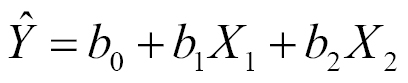# Multiple regression

More often than not, we want to include not just one, but multiple predictors (independent variables) in our predictive models. Luckily, linear regression can easily accommodate us! The technique? Multiple regression.

By giving each predictor its very own beta coefficient in a linear model, the target variable gets informed by a weighted sum of its predictors. For example, a multiple regression using two predictor variables looks like this:Now, instead of estimating two coefficients ( band  b1), we are estimating three: the intercept, the slope of the first predictor, and the slope of the second predictor.

Before explaining ...

Get Data Analysis with R - Second Edition now with the O’Reilly learning platform.

O’Reilly members experience books, live events, courses curated by job role, and more from O’Reilly and nearly 200 top publishers.